# 1. Introduction

The GATE application (Geant4 Application for Emission Tomography) provides a series of tools that allow the collection of data on the interaction of radiation with matter during the simulation, such as the energy deposited and the particles created within a volume, among others. The objective of this work is to evaluate the impact of the size of the dose element in the simulation of the absorbed dose in an attenuating medium in the GATE application, using 99mTc and 18F point sources. An analysis of the influence of the size of the elements of the dose map (canopy) on the absorbed dose was performed, as well as the impact of different font configurations. The results show that a matrix with larger voxels sub-sample the absorbed dose values, especially when closer to the source. Thus, for a more accurate dosimetry it is recommended to use smaller size dosels close to the source. In relation to the 18F simulation, the simulations must be carried out with the source configured as “Fluor18” so that all physical processes are properly considered. It is concluded that the GATE application is a reliable environment for the dose estimation in nuclear medicine images, allowing the investigation and selection of the radiation interaction processes with the most relevant matter for internal dosimetry. Keywords: internal dosimetry; nuclear medicine; GATE; Dose map; Monte Carlo simulation.

GATE (Geant4 Application for Emission Tomography) is a toolkit for the application of Geant4 for Monte Carlo simulation (MC) for emission tomography. It is an open source simulation platform in MC, which incorporates the Geant4 libraries, in a modular, versatile tool package programmable through scripts, for simulating medical images, radiotherapy and dosimetry in the same environment1–3.

The GATE platform allows an accurate description of time-dependent phenomena, such as the movement of detectors, the modeling of breathing and cardiac movements, as well as the kinetics of radiotracers1,2. It allows a more realistic modeling of medical imaging systems, allowing the simulation of raw data of radiation interaction with the patient’s body, even allowing to evaluate the accuracy of tomographic reconstruction algorithms and correction methods2. In addition, GATE provides a series of tools, called actuators, that allow the collection of relevant data during the simulation, such as the energy deposited and the particles created inside a volume, among others. For the generation of the dose map in GATE, the command “dose actor” is used, which stores the dose in Gy in a 3D matrix. In GATE, the smallest volume element (voxel) within the absorbed dose matrix is ​​called the dose element or canopy, and defines the spatial resolution of the dose map. In nuclear medicine, the radioactive source is found inside the patient, resulting in a greater interaction of the radiation emitted with the tissues closest to the source, due to the greater fluency of the radiation. As the number of interactions in the regions close to the source can be greater than the number of interactions with the most distant detectors, the GATE application allows to optimize the irradiation configuration, minimizing the number of simulated stories through changes in the canopy. As the size of the canopy can be defined by the user, it is necessary to evaluate its impact in determining the absorbed dose for different distances. The objective of this work was to carry out an evaluation of the impact of the dose element size in the simulation of the absorbed dose in a spherical attenuator medium, using 99mTc and 18F point sources simulated in the GATE application.

# 2. Materials and Methods

2.1. Modeling Figure 1 shows the first modeled geometry used in this work, which consisted of a point source (99mTc and 18F), in yellow, centered on a 15 cm radius sphere, in red, filled with water. The world, the structure in which physical processes occur, was defined as a cubic box with a 100 cm edge (in cyan). The second geometry used in this work maintained the previous conditions, only increasing the sphere’s radius to 30 cm. The 99mTc simulations were performed with the generation of 2x107 histories (approximately 0.5 mCi), corresponding to the emission of gamma photons of energy 140 keV, in order to allow the comparison of the results with the work carried out by Papadimitroulas5. The 18F source simulation had activity defined as 3.7 MBq (0.1 mCi), corresponding to 3.7x106 stories. In this case, the same number of 99mTc histories was not used, since the 18F emission occurs through the emission of positrons, annihilation and subsequent emission of two collinear gamma photons. The two possible configurations of the 18F source were evaluated: “Fluor18” and “back-to-back”, in 1-second simulations. The following physical interaction libraries were used: (i) Standard, for photoelectric effect (EF), ionization (I), Compton scattering (EC), Bremmstrahlung (B), multiple scattering by Coulomb interactions (MSC), and annihilation of positrons (A); and (ii) Penelope, for spreading Rayleigh (R).

2.2. Influence of Dose Element Size The GATE application allows the matrix in which the absorbed dose is calculated to be defined with variable canopy sizes in x, y and z. In this work, the canopy was defined as a series of small isotropic cubes (voxels). To assess the influence of the size of the dose element, the dimensions of the canopy tested were 0.15 x 0.15 x 0.15 cm3, 0.20 x 0.20 x 0.20 cm3 and 0.30 x 0.30 x 0.30 cm3.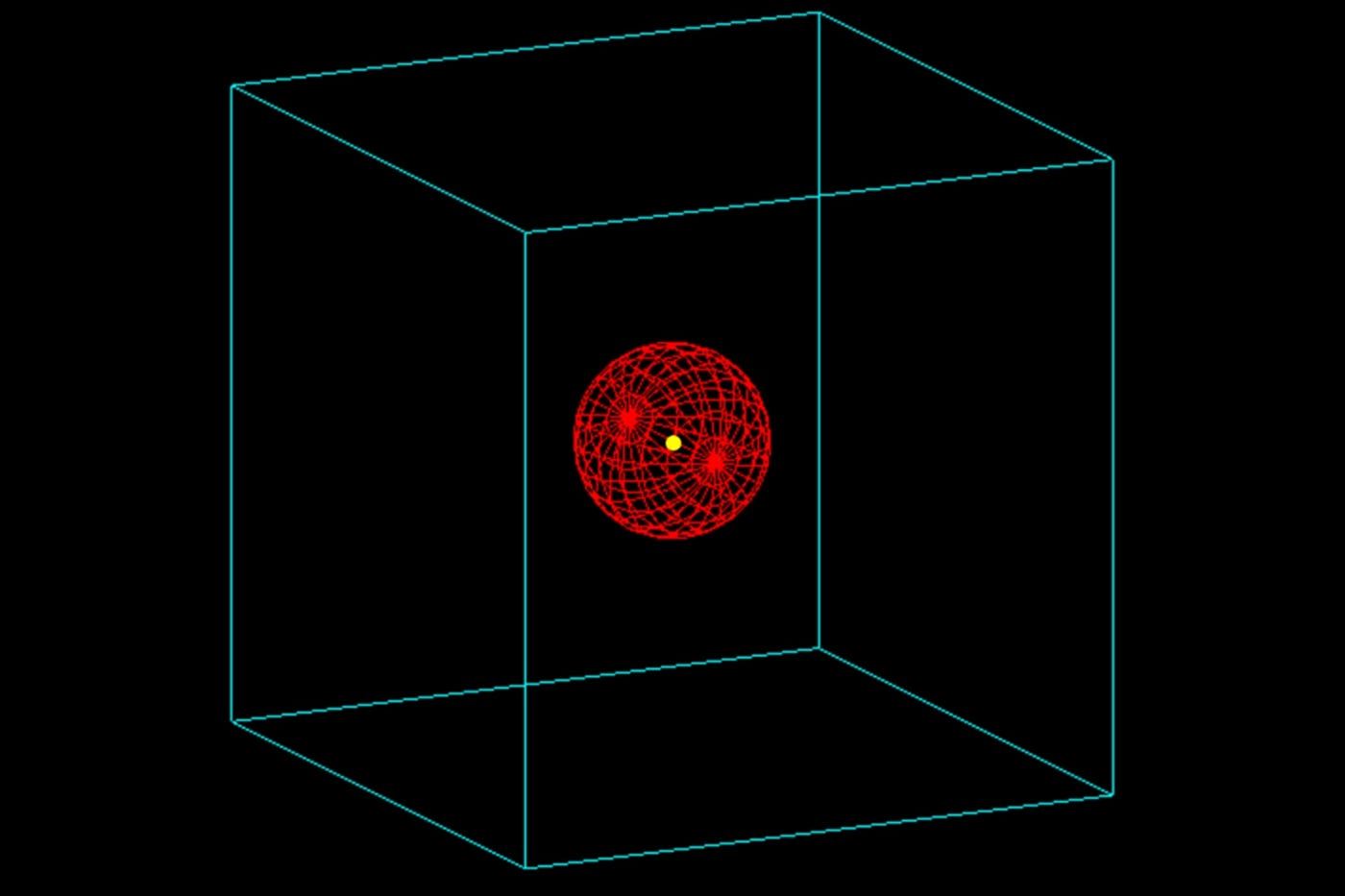Figure 1. Modeled geometry. In cyan color, the simulation world box. In red, the 15 cm radius sphere with water, in which the dose is determined. In the center of the sphere, in yellow, is the point source.

The analysis of the absorbed dose was performed using the dose point kernel or DPK (Dose Point Kernel) technique 6, which consists of determining the value of the energy deposited in each ray of a sphere of known material, homogeneously distributed, due to a centered point source emitting radiation. The dose distribution was calculated in different concentric spheres, generated from the center, as shown in Figure 2.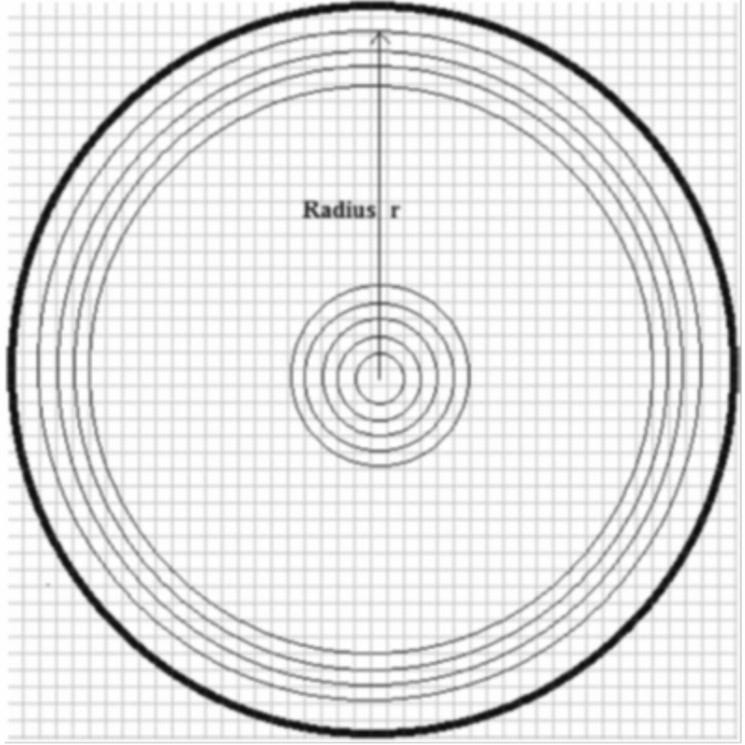Figure 2. 2D representation of the concentric spheres around a centered radioactive source, in which the dose absorbed by the DPK5 method is calculated.

2.3. 18F Font Settings The GATE application allows two font configurations to be simulated for 18F emission, configured with the names: “Fluor18” and “back-toback”. Each type of source obeys the following characteristics: • Fluor18: source configuration in which the energy spectrum of the positrons emitted due to the 18F decay is defined; • Back-to-back: source configuration in which only two photons of annihilation with 180º collinear angles are generated. The two configurations of the 18F source were simulated separately for analysis of positron interaction and subsequent emission of collinear gamma photons, and, separately, only collinear gamma photons. The canopy size used to construct the dose map for comparison between the two source models was 0.15 x 0.15 x 0.15 cm3. For a more detailed analysis of the physical processes of radiation interaction with matter using the source configured as “Fluor18”, simulations of a point source of 37 MBq (0.1 mCi) were performed, using all combinations of physical process configurations. described in subsection 2.1. For computational time optimization purposes, the canopy size used was 0.30 x 0.30 x 0.30 cm3.

3.1 Influência do Tamanho do Elemento de Dose 3.1.1 Fonte de 99mTc A Figura 3 mostra os valores de dose na esfera de água devido a uma fonte pontual centrada de 99mTc, normalizada pelo valor máximo de dose, para cada dosel simulado, em função da distância da fonte, para os diferentes dosels. As cores representam as diferentes dimensões de dosel simulados: 0,15 x 0,15 x 0,15 cm3 (ciano), 0,20 x 0,20 x 0,20 cm3 (verde) e 0,30 x 0,30 x 0,30 cm3 (vermelho).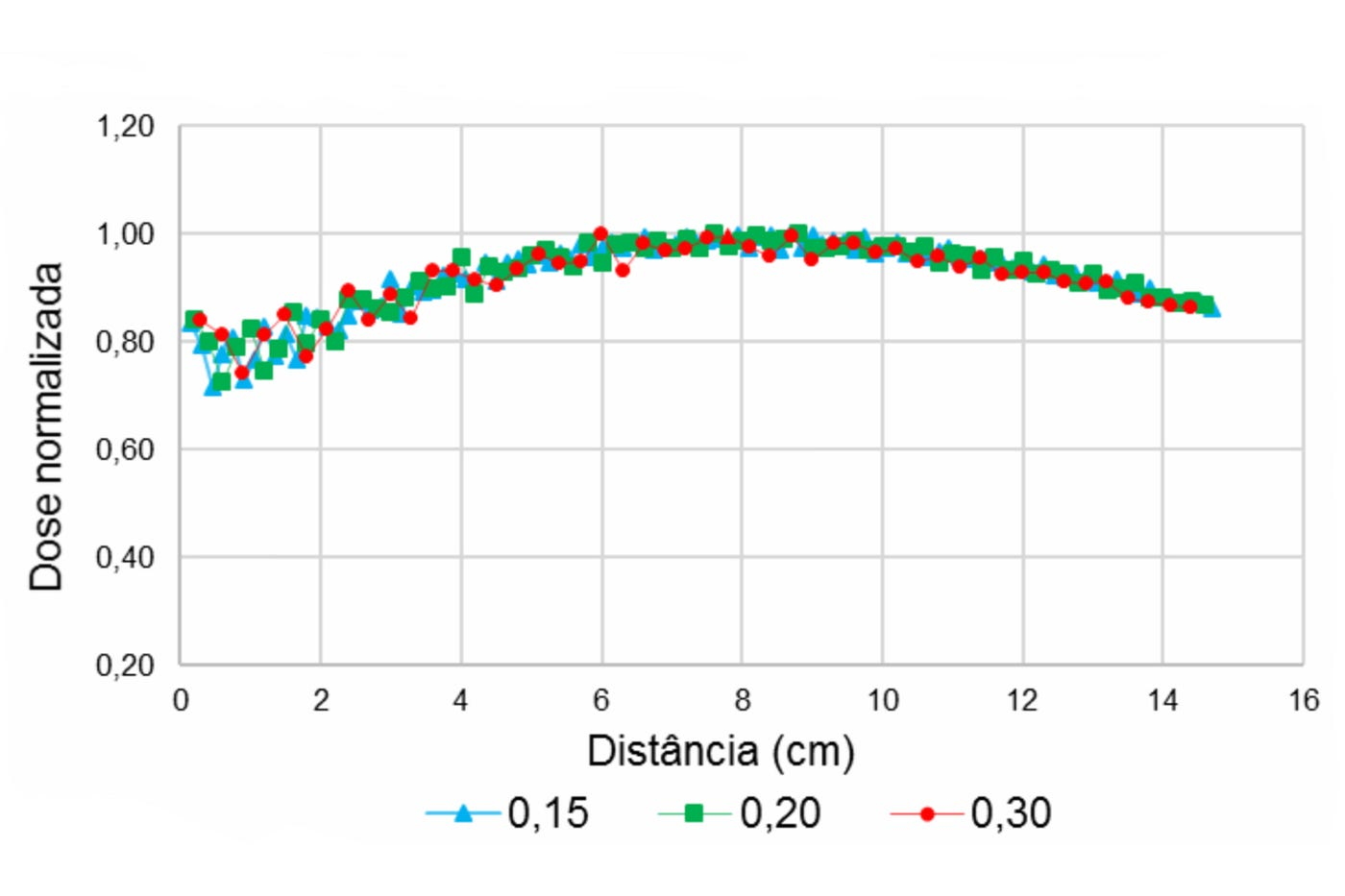Figure 3. Absorbed dose normalized to the maximum dose value, for the 99mTc source, depending on the distance from the source, for different dosage sizes.

For purposes of comparison with the results presented by Papadimitroulas5 for the simulation of a 99mTc source, a simulation was performed with a water sphere with a radius of 30 cm, canopy of 0.30 cm. Figure 4 shows the result of the dose normalized to the maximum value.

## 3.1.2 18F

source Figure 5 shows the absorbed dose values for the 18F source configured as “Fluor18”, for each canopy size evaluated (a) and the dose normalized to the maximum value (b). The colors represent the simulated canopies of different dimensions: 0.15 x 0.15 x 0.15 cm3 (cyan) 0.20 x 0.20 x 0.20 cm3 (green) and 0.30 x 0.30 x 0 , 30 cm3 (red).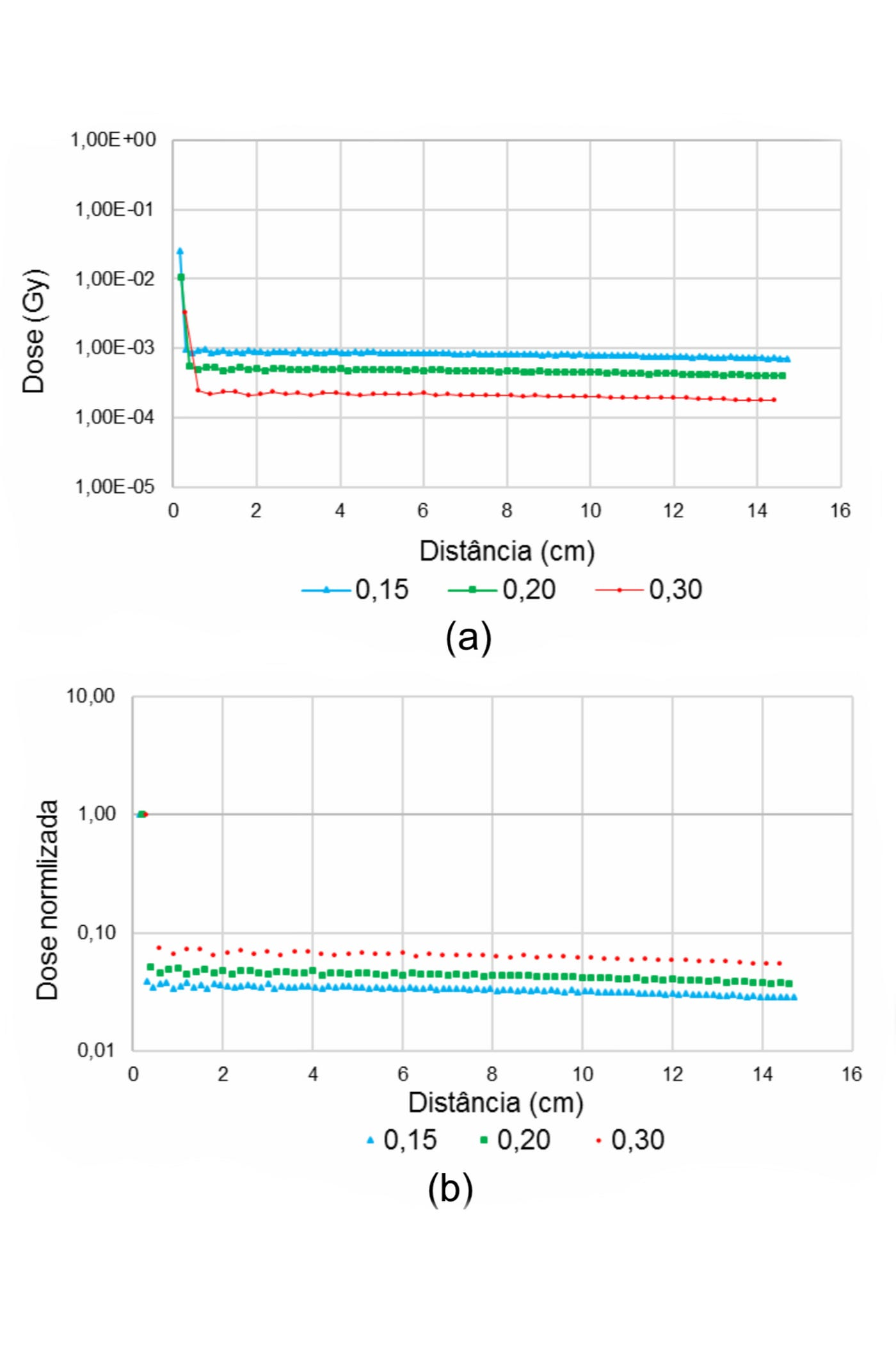Figure 5. Doses absorbed in different doses for the 18F source, depending on the distance from the source. In (a) the absolute doses are presented for each canopy size. In (b), the normalized dose. The y-axis is on a logarithmic scale.

For a better visualization of the behavior of the absorbed dose curve for the source configured as “Fluor18”, a simulation was performed with a 30 cm radius water sphere, 0.30 cm canopy. Figure 6 shows in (a) the normalized dose with the first dose point and in (b), without the first point, showing the behavior of the curve over the distance.

## 3.2 Interaction of positron emitting sources (18F) with matter

Figure 7 shows the absorbed doses, in Gy, as a function of the distance from the center of the sphere, with a 0.15 cm canopy, when using the different types of configuration of the sphere. 18F font in the GATE application: “Fluor18” and “back-to-back”. To evidence the differences, a logarithmic dose scale was used. The red arrow highlights the first dose deposition point, which appears only when the “Fluor 18” configuration is used.

The percentage difference between the dose in the simulations with the two 18F source configurations in Figure 7 is 23%. Disregarding the dose for the dose closest to the source, the percentage difference between the doses with the two settings of 18F drops to 0.45%.

For the configured source “Fluor18”, the following physical processes did not show energy deposition in the sphere: those associated with annihilation individually and associated with the Bremsstrahlung (B), Rayleigh scattering (R) and MSC processes. Physical processes that combined annihilation and ionization had energy deposition only in the first central sphere of the DPK, where the 18F source is located.

The process of ionization of the medium made a contribution of 23% of the total dose in the sphere. At the first dose point, this contribution is greater, with 92.6% of the total dose found. In order to verify the effective contribution of Compton scattering to the deposition of energy, this physical process was associated with the process of annihilation and ionization.

The Error! Reference source not found. shows, in the green curve, the dose when only the positron annihilation process associated with Compton scattering occurs. In black, the dose distribution is found for when all the physical processes of interaction of radiation with matter occur, for comparison purposes.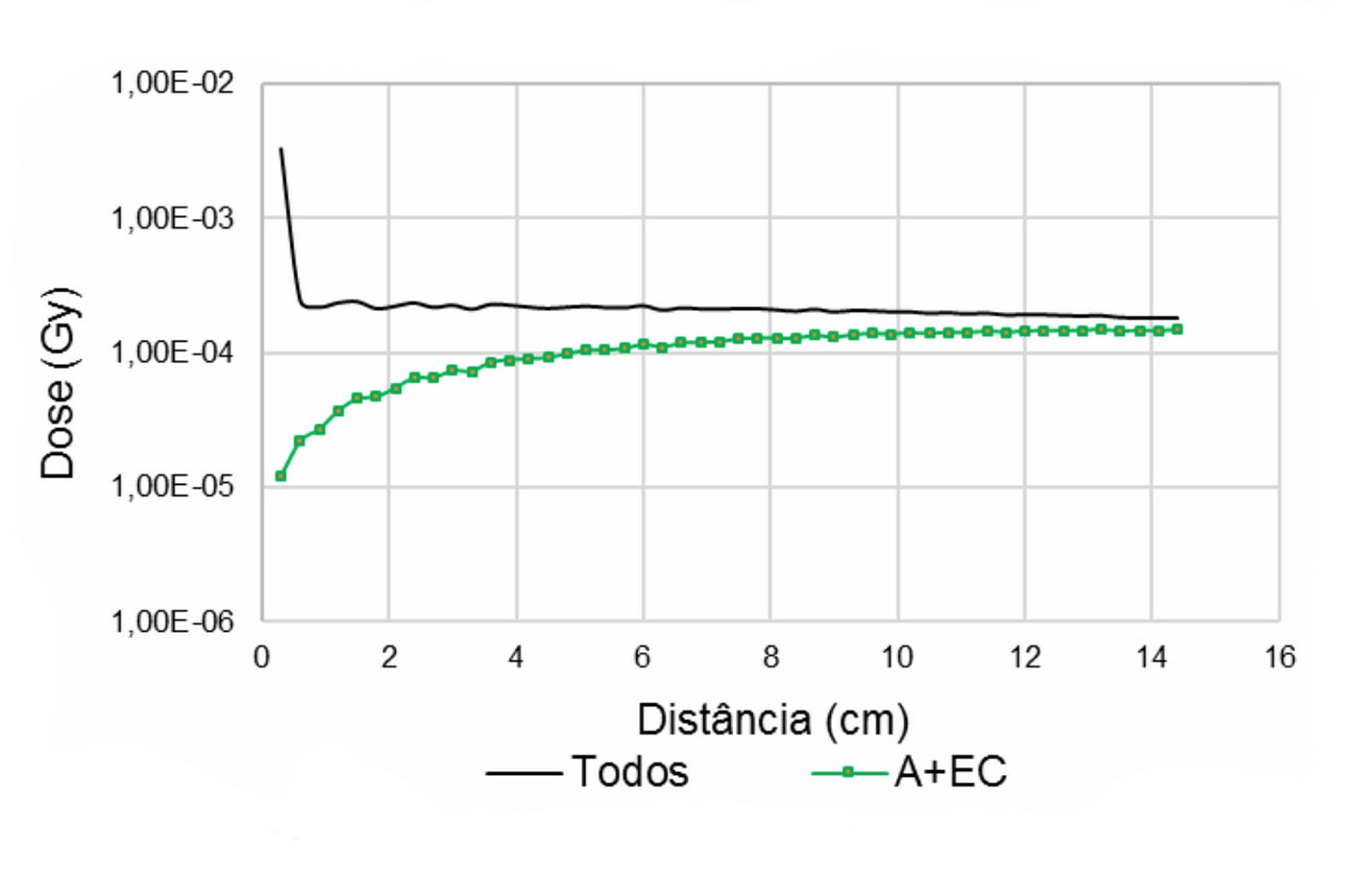Figure 8. Dose absorbed due to all processes (black line) for the “Fluor18” source. In green, energy deposition process without ionization of the medium and only with Compton scattering (EC) enabled.

The dose deposited due to Compton scattering after annihilation represents 40.4% of the contribution to energy deposition, mainly after half the sphere radius (7.5 cm). When the processes of annihilation and Compton scattering are associated with ionization, the individual contribution of EC increases to 98.6% of the total dose. When the dose contribution is simulated only with a process of interaction of radiation without charge with the matter (A + EC + EF + R), it is obtained that the Rayleigh scattering (R) contributes about 0.004% of the dose.

The photoelectric effect represents 0.004%. By associating the process of ionization of the medium with Compton scattering and photoelectric effect, EF represents 1.4% of the deposited dose. The photoelectric effect, associated with other physical processes of interaction of radiation with matter, was simulated with and without the participation of Compton scattering.

Without Compton scattering, doses due to the photoelectric effect, after only annihilation, and annihilation and ionization of the positron emitted by the source in the total dose they were 0.002% and 23%, respectively.

# 4. Discussion

## 4.1.1 Source of 99mTc

Similar behavior is observed for the normalized dose as a function of distance, for all dosages used for 99mTc, after a distance approximately equal to half the radius of the sphere (Figures 3 and 4). Thus, the size of the canopy does not significantly affect the dose calculation for the source of gamma radiation.

However, normalized dose values ​​show greater variability in the vicinity of the source, for all canopy sizes, especially in the first 4 cm. This behavior is due to the small number of elements that compose the dose calculation for spherical shells with a small radius.

This effect of variation due to sampling disappears as the spherical shells of greater radius, which have a greater number of elements. Such dose results are compatible with those found by other researchers in simulations of radionuclide emission with the GATE4,5 application. The matrix with larger dosels sub-sample the dose values, mainly in the positions closest to the source.

Thus, for more accurate dosimetry, it is recommended to use smaller size dosels at the points closest to the source. In case it is necessary to decrease the computational time of the simulation, it is suggested to use variable canopy sizes, with a larger canopy size for points more distant from the source.

## 4.1.2 18F Source Settings

A similar behavior of the absorbed dose is observed in all the dosages used for the 18F simulation, configured for “Fluor18”, which considers the positron emission (Figure 5). Due to the strong interaction of positrons with the medium, there is a high deposition of energy before the first centimeter of distance from the source.

However, the values ​​of the dose absorbed in the water sphere for the 18F source are significantly different for different dosage sizes. Among the hypotheses to explain this behavior is the fact that the dose in each canopy is calculated by the average of interactions that occurred within the volume.

Thus, as the number of interactions is approximately the same within the dimensions of the evaluated dosels, the lower the volume of the canopy, the greater the number of interactions per unit volume, generating a higher total dose value in the smallest canopy, as shown Figure 5 (a).

This effect is highlighted by the fact that this source is configured with the emission of positrons, which generate a high dose in the first dose. Thus, the dose at the first point close to the source is higher for the 0.15 x 0.15 x 0.15 cm3 canopy than for the 0.20 x 0.20 x 0.20 cm3 canopy, and for the 0.30 x 0.30 0.30 cm3 canopy (Figure 5 (a)).

Larger studies should be carried out to assess such assumptions. But, it can be assumed that for a better sampling, the smallest canopy should be used in simulations close to the source.

In addition, the different settings of the 18F source in the GATE application show a great impact on the dose. The source configured as “Fluor18” shows that a high dose deposition is carried out close to the source (Figure 6 a), resulting from the interaction of the positrons and a slower energy deposition behavior from the second concentric sphere (Figure 6 b) , resulting from the interaction with gamma radiation of annihilation.

The positrons, being charged particles, interact with the medium quickly depositing their energy mainly by ionization at the points closest to the source, which is consistent with the average range of the positrons (Rmean = 0.6 mm and Rmax = 2.4 mm) 7. The dose due to ionization, after annihilation, is deposited before the first 2 cm away from the source, which is shown in the first point of the red curve (red arrow) in Figure 7. In the “back-toback” configuration, this deposition effect high dose close to the source is not observed in the simulation results, as this configuration considers only the emission of annihilation photons.

The percentage difference between the total dose in the two 18F source configurations is 23%. Despite this difference, the use of the configured back-toback source for the 18F can be used to simulate positron emission tomography images or to estimate energy deposition in target tissues distant from the source tissue, without significantly impairing the results, since the difference drops to 0.45% for distances greater than a few millimeters from the source. The dose deposition in the source tissues, however, must take into account this dose contribution due to the positrons.

The dose in the vicinity of the source is basically due to the physical process of ionization, while more distant points, the predominant process is the Compton scattering, agreeing with the findings for the energy range of the collinear photons emitted and the atomic number of the material used in the simulation8.

The initial studies carried out with the GATE application with simple attenuating sources and objects showed that there is a possibility to determine the internal dose using different sized canopies in the simulations. As for the simulation of positron emitting sources in the GATE application, it is suggested to use the pre-configured source as “Fluor18” so that the simulation includes the dose contributions of both the interaction of positrons and gamma photons resulting from annihilation, particularly in internal dosimetry studies.

# 5. Conclusions

This study showed that the size of the voxel to be used in dosimetry by the DPK technique presents the biggest differences in the absorbed dose when the evaluated points are closer to the source, both for the 99mTc source as well as for the 18F source. The influence of the size of the canopy becomes negligible at greater distances. For a more accurate dosimetry it is suggested that the size of the canopy be as small as possible.

In order to make the simulations feasible and reduce the computational time, it is suggested to use different sizes of dosels for different distances from the source. Despite suggesting the choice of the source type for 18F as “Fluor18”, for the purpose of studying the interaction of radiation with matter, the configuration of the source as back-to-back, which simulates only the emission of collinear photons, can be of great interest for the simulation of images and for the dosimetry of organs distant from the source organ.

As for the behavior of the physical processes of interaction of radiation with matter in the deposition of energy in water by an 18F source, it is noticed that after strong initial interaction of the positron with the medium by ionization, Compton scattering becomes predominant in the interaction processes of radiation with matter.

Among the limitations of this study, the errors generated by the statistical uncertainties intrinsic to the Monte Carlo simulation approach stand out, which is strongly dependent on the number of simulated events. For future work, we intend to increase the number of simulated stories, requiring an effort to parallelize computer simulations. Additionally, it is intended to carry out an analysis of the influence of the size of the canopy in regions closer to the source in determining the absorbed dose.

# 6. References

1. ASSIÉ, K. et al. Monte Carlo simulation in PET and SPECT instrumentation using GATE. Nuclear Instruments and Methods in Physics Research Section A: Accelerators, Spectrometers, Detectors and Associated Equipment, v. 527, n. 1–2, p. 180–189, jul. 2004. 2. LOUDOS, George K. Monte Carlo simulations in Nuclear Medicine. In: GRANJA, Carlos; LEROY, Claude; STEKL, Ivan (Ed.). AIP Conference Proceedings. AIP, 2007. p. 147–150. 3. SARRUT, D. et al. A review of the use and potential of the GATE Monte Carlo simulation code for radiation therapy and dosimetry applications. Medical Physics, v. 41, n. 6, p. 064301, jun. 2014. 4. LOUDOS, G. et al. A radionuclide dosimetry toolkit based on material specific Monte Carlo dose kernels. Nuclear Medicine Communications, v. 30, 2009. 5. PAPADIMITROULAS, Panagiotis et al. Photon dose kernels dataset for nuclear medicine dosimetry, using the GATE Monte Carlo toolkit. In: Nuclear Science Symposium and Medical Imaging Conference (NSS/MIC), 2011 IEEE. IEEE, 2011. p. 1390–1394. 6. PAPADIMITROULAS, P.; LOUDOS, G. K.; NIKIFORIDIS, G.; KAGADIS, G.C. A dose point kernel database using GATE Monte Carlo simulation toolkit for nuclear medicine applications: comparison with other Monte Carlo codes. Medical Physics, v. 39, n. 8, p. 5238–5247, Aug. 2012. 7. ALVA-SÁNCHEZ, H. et al. Positron range in tissue-equivalent materials: experimental microPET studies. Physics in Medicine & Biology, v. 61, n. 17, p. 6307, 2016. 8. ATTIX, Frank Herbert. Introduction to radiological physics and radiation dosimetry. John Wiley & Sons, 2008.

--

--